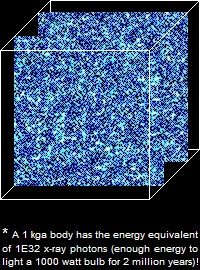Mass/energy in the universe
The term mass/energy in the two premises reflects the fact that all mass is comprised of energy according to Einstein's relationship e=m·c, where energy (e) is in joules, mass (m) is in kilograms, and cis 9E16 (m/s). In the qm view, where the unit of velocity is ca and the unit of mass and energy is the absolute kilogram (kga), the mass/energy relationship is as follows.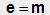In the qm view, a body's mass m depends on the quantity and the energy of the energy quanta in the body. And according to orthodox theory, the energy e of an energy quantum is a function of its oscillation frequency (f) and the Planck constant (h) as follows.Combining the above equations we get m=h·f, showing that a body's mass depends on the oscillation frequencies of the energy quanta in the body. We will show that the oscillation frequencies of photons moving within a body depend on the body's velocity va through the qm, and that the average energy of photons increases as va increases. It is assumed that a body's absolute velocity affects the oscillation frequencies and energies of all energy quanta in the body to the same extent photons are affected. We will see that this assumption is consistent with the known relationship between a body's observed velocity and the body's observed mass.

Due to the huge number of energy quanta moving within a body* and the great complexity of the system of energy in a body, the energy quanta are assumed to be moving in all directions about equally. It can be expected that the average energy of a body's energy quanta moving parallel to a given plane will be about the same as the average energy of the body's energy quanta moving parallel to any other plane. On the next page we model energy quanta moving in a single plane that is representative of an entire body and we analyze how the energies of the energy quanta change when the body's velocity through the qm changes.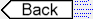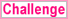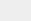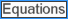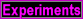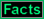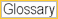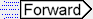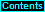To page: 1 2 3 3a 4 5 6 7 8 9 10 11 12 13 14 15 16 17 18 19 20 21 22 23 24 25 26 27 28 29 29a 30 31 32